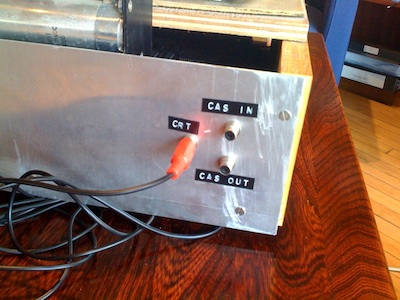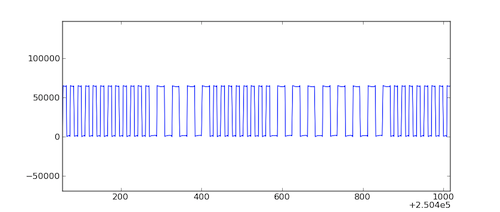# Dabeaz

Dave Beazley's mondo computer blog. [ homepage | archive ]

## Decoding Superboard II Cassette Audio Using Python 3, Two Generators, and a Deque

Welcome to the second installment of using Python to encode/decode cassette audio data for use with my resurrected Superboard II system. Last time, I talked about the problem of encoding text files into WAV audio files for uploading via the Superboard cassette input. In this post, I explore the opposite problem--namely using Python to decode WAV audio files recorded from the cassette output port back into the transmitted byte stream--in essence, writing a Python script that performs the same function as a modem.The cassette ports of my Superboard II

Although decoding audio data from the cassette output sounds like it might be a tricky exercise involving sophisticated signal processing (e.g., FFTs), it turns out that you can easily solve this problem using nothing more than a few built-in objects (bytearrays, deques, etc.) and a couple of simple generator functions. In fact, it's a neat exercise involving some of the lesser known, but quite useful data processing features of Python. Plus, it seems like a good excuse to further bang on the new Python 3 I/O system. So, let's get started.

Audio Format

In my earlier post, I described how the format used for cassette recordings is the Kansas City Standard (KCS). The encoding is really simple--8 cycles at 2400 HZ represent a 1-bit and 4 cycles at 1200 HZ represent a 0-bit. Individual bytes are encoded with 1 start bit (0) and two stop bits (1s). Here is a plot that shows some waveforms from a fragment of recorded audio.It is important to stress that this encoding is intentionally simple--designed to operate on systems of its era (1970s) and to be resistant to all sorts of problems associated with cassette tapes. For example, noise, low-fidelity, variations in tape playback speed, etc. Needless to say, it's not especially fast. Encoding a single byte of data requires 11 bits or 88 cycles of a 2400 HZ wave. If you do the math, that works out to about 27 bytes per second or 300 baud.

A Decoding Strategy (Big Picture)

KCS decoding is almost entirely based on counting cycles of two different wave frequencies. That is, to decode the data we simply sample the audio data and count the number of zero-crossings. At a high level, decoding a single bit works as follows:

• Read a sample of N audio frames where N represents the number of frames required to represent an entire bit (8 cycles at 2400 Hz).
• Count the number of zero crossings found in the sample.
• If the the number of crossings is near 16, then it represents a 1.
• If the number of crossings is near 8, then it represents a 0.

From bits, it's relatively simple to make the transition to bytes. You simply have to recognize the start bit and sample the next 8 bits as data bits to form a byte.

Deconstructing a WAV File to Sign Bits

Python has a module wave that can be used to read WAV files. Here is an example of opening a WAV file and obtaining some useful metadata about the recorded audio.

```>>> import wave
>>> wf = wave.open("osi_sample.wav")
>>> wf.getnchannels()
2
>>> wf.getsampwidth()
2
>>> wf.getframerate()
44100
>>>
```

In the above example, the WAV file is a 44100Hz stereo recording using 16-bit (2 byte) samples.

For our decoding, we are only interested in counting the number of zero-crossings in the audio data. For a 16-bit WAV file, the "zero" is represented by a sample value of 2**15 (32768). A "positive" wave sample has a value greater than 2**15 whereas a "negative" wave sample has a value less than that. Conveniently, this determination can be made by simply stripping all sample data away except for the most significant bit.

Here is a generator function that takes a sequence of WAV audio data and reduces it to a sequence of sign bits.

```# Generate a sequence representing sign bits
def generate_wav_sign_bits(wavefile):
samplewidth = wavefile.getsampwidth()
nchannels = wavefile.getnchannels()
while True:
if not frames:
break

# Extract most significant bytes from left-most audio channel
msbytes = bytearray(frames[samplewidth-1::samplewidth*nchannels])

# Emit a stream of sign bits
for byte in msbytes:
yield 1 if (byte & 0x80) else 0
```

This generator works by reading a chunk of raw audio frames and using an extended slice frames[samplewidth-1::samplewidth*nchannels] to extract the most significant byte from each sample of the left-most audio channel. The result is placed into a bytearray object. A bytearray stores a sequence of bytes (like a string), but has the nice property that the stored data is presented as integers instead of 1-character strings. This makes it easy to perform numeric calculations on the data. The yield 1 if (byte & 0x80) else 0 simply yields the most significant bit of each byte.

The resulting output from this generator is simply a sequence of sign bits. For example, the output will look similar to this:

```>>> import wave
>>> wf = wave.open("sample.wav")
>>> for bit in generate_wav_sign_bits(wf):
...     print(bit,end="")
...
11111111000000000111111111000000000011111111100000000011111111110000000001111111
11000000000011111111100000000011111111110000000001111111110000000000111111111000
00000011111111110000000001111111110000000000111111111000000000111111111100000000
01111111110000000000111111111000000000111111111100000000011111111100000000001111
...
```

From Sign Bits to Sign Changes

Although a sequence of wave sign bits is interesting, it's not really that useful. Instead, we're really more interested in zero-crossings or samples where the sign changes. Getting this information is actually pretty easy--simply compute the exclusive-or (XOR) of successive sign bits. If you do this, you will always get 0 when the sign stays the same or a value 0x80 when the sign flips. Here is a modified version of our generator function.

```# Generate a sequence representing changes in sign
def generate_wav_sign_change_bits(wavefile):
samplewidth = wavefile.getsampwidth()
nchannels = wavefile.getnchannels()
previous = 0
while True:
if not frames:
break

# Extract most significant bytes from left-most audio channel
msbytes = bytearray(frames[samplewidth-1::samplewidth*nchannels])

# Emit a stream of sign-change bits
for byte in msbytes:
signbit = byte & 0x80
yield 1 if (signbit ^ previous) else 0
previous = signbit
```

This slightly modified generator now produces a sequence of data with sign change pulses in it similar to this:

```>>> import wave
>>> wf = wave.open("sample.wav")
>>> for bit in generate_wav_sign_change_bits(wf):
...     print(bit,end="")
...
00000000100000000100000000100000000010000000010000000010000000001000000001000000
00100000000010000000010000000010000000001000000001000000001000000000100000000100
00000010000000001000000001000000001000000000100000000100000000100000000010000000
01000000001000000000100000000100000000100000000010000000010000000010000000001000
...
```

Bit Sampling

At this point, the WAV file has been deconstructed into a sequence of sign changes. Now, all we have to do is sample the data and count the number of sign changes. To do this, use a deque and some clever iterator tricks. Here is some code:

```from itertools import islice
from collections import deque

# Base frequency (representing a 1)
BASE_FREQ = 2400

# Generate a sequence of data bytes by sampling the stream of sign change bits
def generate_bytes(bitstream,framerate):

# Compute the number of audio frames used to encode a single data bit
frames_per_bit = int(round(float(framerate)*8/BASE_FREQ))

# Queue of sampled sign bits
sample = deque(maxlen=frames_per_bit)

# Fill the sample buffer with an initial set of data
sample.extend(islice(bitstream,frames_per_bit-1))
sign_changes = sum(sample)

# Look for the start bit
for val in bitstream:
if val:
sign_changes += 1
if sample.popleft():
sign_changes -= 1
sample.append(val)

# If a start bit detected, sample the next 8 data bits
if sign_changes <= 9:
byteval = 0
if sum(islice(bitstream,frames_per_bit)) >= 12:
yield byteval
# Skip the final two stop bits and refill the sample buffer
sample.extend(islice(bitstream,2*frames_per_bit,3*frames_per_bit-1))
sign_changes = sum(sample)
```

This code might require some study, but the concept is simple. A sample deque (the sample variable) is created, the size of which corresponds to the number of audio frames needed to represent a single data bit. It might be a little known fact, but if you create a deque with a maxlen setting, it turns into a kind of shift register. That is, new items added at the end will automatically cause old items to fall off the front if the length is exceeded. It is also very fast.

Getting back to our algorithm, audio data is pushed into this deque and the number of sign changes updated. If no data is being transmitted, the number of sign changes in the sample will hover around 16. However, if a start-bit is encountered, the number of sign changes in the sample will drop to around 8. In our code, this is detected by checking for 9 or fewer sign changes in the sample. Keep in mind that we don't really know when the start bit will appear--thus, the code proceeds frame-by-frame until the number of sign changes drops to a sufficiently low value. Once the start bit is detected, data bits are quickly sampled, one after the other, to form a complete byte. After the data bits are sampled, the two stop bits are skipped and the sample buffer refilled with the next potential start bit.

Does it Work?

Hell yes it works. Here is a short test script that ties it all together:

```if __name__ == '__main__':
import wave
import sys
if len(sys.argv) != 2:
print("Usage: %s infile" % sys.argv,file=sys.stderr)
raise SystemExit(1)

wf = wave.open(sys.argv)
sign_changes = generate_wav_sign_change_bits(wf)
byte_stream  = generate_bytes(sign_changes, wf.getframerate())

# Output the byte stream
outf = sys.stdout.buffer.raw
while True:
buffer = bytes(islice(byte_stream,80))
if not buffer:
break
outf.write(buffer)
```

If we run this program on the osi_sample.wav file, we get the following output (which is exactly what it should be):

```bash-3.2\$ python3 kcs_decode.py osi_sample.wav

10 FOR I = 1 TO 1000
20 PRINT I;
30 NEXT I
40 END
OK
bash-3.2\$
```

That's pretty nice--two relatively simple generator functions and some basic data manipulation on deques has turned the audio stream into a stream of bytes.

One thing that's not shown above is the embedded NULLs related to newline handling. You can see them if you do this:

```bash-3.2\$ python3 kcs_decode.py osi_sample.wav | cat -e
^M^@^@^@^@^@^@^@^@^@^@\$
^M^@^@^@^@^@^@^@^@^@^@\$
10 FOR I = 1 TO 1000^M^@^@^@^@^@^@^@^@^@^@\$
20 PRINT I;^M^@^@^@^@^@^@^@^@^@^@\$
30 NEXT I^M^@^@^@^@^@^@^@^@^@^@\$
40 END^M^@^@^@^@^@^@^@^@^@^@\$
OK^M^@^@^@^@^@^@^@^@^@^@\$
bash-3.2\$
```

How well does it work?

To test this decoding process, I recorded various audio samples directly from my Superboard using Audacity on my Mac. I used different sampling frequencies ranging from 8000 Hz to 48000 Hz. For all of the samples, the decoding process worked exactly as expected, producing no observable decoding errors.

Decoding 5788 bytes of transmitted test data from 47 Mbyte WAV file of 48 KHz stereo samples takes about 5.7 seconds on my Macbook (2.4 Ghz Intel Core Duo) for a baud rate of about 11000--more than 35 times faster than the Superboard can actually send it. Decoding the same data recorded in a 7.3 Mbyte WAV file with 8 KHz stereo samples takes about 0.97 seconds for a baud rate of about 65000 (Note: these baud rates are based on 11 bits of encoding for every data byte).

Although I could work to make the script run faster, it is already plenty fast for my purposes. Moreover, the generator-based approach means that they really aren't limited by the size of the input WAV files.

Final Words

If you are interested in the final script, you can find it in the file kcs_decode.py. Although I've now written scripts to encode and decode Superboard II cassette audio data, this is the hardly the last word. Stay tuned (evil wink ;-).

Footnote

If you're going to try any of this code, make sure you're using Python-3.1.2 or newer. Earlier versions of Python 3 seem to have buggy versions of the wave module.

## ArchivesSubscribe to Posts [Atom]